# Demo#9

Open
opened this issue Feb 28, 2019 · 45 comments
Open

# Demo #9

opened this issue Feb 28, 2019 · 45 comments

### MassyMeniche commented Feb 28, 2019

 First of all thank you for the great work. I'm currently trying to set up a demo of the estimator but run into some issues in the post-processing stage (the network output is a B x 17 x 128 x 128 for 512x512 images) Are planning to release any helper functions for post-processing the output to a key-points ? Many thanks The text was updated successfully, but these errors were encountered:

### leoxiaobin commented Feb 28, 2019

 When I am free, I will add a demo for inference. For your issue, you can read our code at https://github.com/leoxiaobin/deep-high-resolution-net.pytorch/blob/master/lib/core/inference.py, which include how to get the final prediction with the heatmaps.

### lucasjinreal commented Mar 18, 2019

 @MassyMeniche @leoxiaobin With a quick dive into codes, find a function to get max preds: ``````heatmaps = out.detach().cpu().numpy() preds, maxvals = get_max_preds(heatmaps) `````` Which `out` is simply raw ouput of network. the preds shape is Bx17x2. I suppose it's 17 keypoints coordinates? But when I draw it it does not seems right:What does that function gets? How to get the final keypoints coordinates finally?

### wait1988 commented Apr 2, 2019

 @jinfagang Have you solved this problem yet?

### leoxiaobin commented Apr 9, 2019

 @jinfagang ， after you get the preds, you should also need to project the coordinates to the original image, using the function at https://github.com/leoxiaobin/deep-high-resolution-net.pytorch/blob/master/lib/core/inference.py#L49****

### wait1988 commented Apr 11, 2019

 @leoxiaobin What does the center and scale mean?

### njustczr commented Apr 14, 2019

 @jinfagang ， after you get the preds, you should also need to project the coordinates to the original image, using the function at https://github.com/leoxiaobin/deep-high-resolution-net.pytorch/blob/master/lib/core/inference.py#L49**** what does the center and scale mean?...

### lucasjinreal commented Apr 15, 2019

 @njustczr After a digging in, I think it's the object detection box.. which means you should do object detection first..

### njustczr commented Apr 15, 2019

 @njustczr After a digging in, I think it's the object detection box.. which means you should do object detection first.. center:bbox center? scale: the ratio of (width / height) ?

### njustczr commented Apr 16, 2019

 @njustczr After a digging in, I think it's the object detection box.. which means you should do object detection first.. get_max_preds() performs better than get_final_preds()?... scale=height/200.0

### gireek commented Jul 22, 2019

 I have the same question. Please share how did you get keypoints on your own data.

### Ixiaohuihuihui commented Aug 25, 2019 • edited

 By refecence this code[https://github.com/microsoft/human-pose-estimation.pytorch/issues/26#issuecomment-447404791], I can get good result. Make a file in the tools folder, and name it as "demo.py" ``````from __future__ import absolute_import from __future__ import division from __future__ import print_function import argparse import os import pprint import torch import torch.nn.parallel import torch.backends.cudnn as cudnn import torch.optim import torch.utils.data import torch.utils.data.distributed import torchvision.transforms as transforms import _init_paths from config import cfg from config import update_config from core.loss import JointsMSELoss from core.function import validate, get_final_preds from utils.utils import create_logger from utils.transforms import * import cv2 import dataset import models import numpy as np def parse_args(): parser = argparse.ArgumentParser(description='Train keypoints network') # general parser.add_argument('--cfg', help='experiment configure file name', default='experiments/mpii/hrnet/w32_256x256_adam_lr1e-3.yaml', type=str) parser.add_argument('opts', help="Modify config options using the command-line", default=None, nargs=argparse.REMAINDER) parser.add_argument('--img-file', help='input your test img', type=str, default='') # philly parser.add_argument('--modelDir', help='model directory', type=str, default='') parser.add_argument('--logDir', help='log directory', type=str, default='') parser.add_argument('--dataDir', help='data directory', type=str, default='') parser.add_argument('--prevModelDir', help='prev Model directory', type=str, default='') args = parser.parse_args() return args def _box2cs(box, image_width, image_height): x, y, w, h = box[:4] return _xywh2cs(x, y, w, h, image_width, image_height) def _xywh2cs(x, y, w, h, image_width, image_height): center = np.zeros((2), dtype=np.float32) center = x + w * 0.5 center = y + h * 0.5 aspect_ratio = image_width * 1.0 / image_height pixel_std = 200 if w > aspect_ratio * h: h = w * 1.0 / aspect_ratio elif w < aspect_ratio * h: w = h * aspect_ratio scale = np.array( [w * 1.0 / pixel_std, h * 1.0 / pixel_std], dtype=np.float32) if center != -1: scale = scale * 1.25 return center, scale def main(): args = parse_args() update_config(cfg, args) logger, final_output_dir, tb_log_dir = create_logger( cfg, args.cfg, 'valid') logger.info(pprint.pformat(args)) logger.info(cfg) # cudnn related setting cudnn.benchmark = cfg.CUDNN.BENCHMARK torch.backends.cudnn.deterministic = cfg.CUDNN.DETERMINISTIC torch.backends.cudnn.enabled = cfg.CUDNN.ENABLED model = eval('models.'+cfg.MODEL.NAME+'.get_pose_net')( cfg, is_train=False ) if cfg.TEST.MODEL_FILE: logger.info('=> loading model from {}'.format(cfg.TEST.MODEL_FILE)) model.load_state_dict(torch.load(cfg.TEST.MODEL_FILE), strict=False) else: model_state_file = os.path.join( final_output_dir, 'final_state.pth' ) logger.info('=> loading model from {}'.format(model_state_file)) model.load_state_dict(torch.load(model_state_file)) model = torch.nn.DataParallel(model, device_ids=cfg.GPUS).cuda() # define loss function (criterion) and optimizer criterion = JointsMSELoss( use_target_weight=cfg.LOSS.USE_TARGET_WEIGHT ).cuda() # Loading an image image_file = args.img_file data_numpy = cv2.imread(image_file, cv2.IMREAD_COLOR | cv2.IMREAD_IGNORE_ORIENTATION) if data_numpy is None: logger.error('=> fail to read {}'.format(image_file)) raise ValueError('=> fail to read {}'.format(image_file)) # object detection box box = [450, 160, 350, 560] c, s = _box2cs(box, cfg.MODEL.IMAGE_SIZE, cfg.MODEL.IMAGE_SIZE) r = 0 trans = get_affine_transform(c, s, r, cfg.MODEL.IMAGE_SIZE) input = cv2.warpAffine( data_numpy, trans, (int(cfg.MODEL.IMAGE_SIZE), int(cfg.MODEL.IMAGE_SIZE)), flags=cv2.INTER_LINEAR) transform = transforms.Compose([ transforms.ToTensor(), transforms.Normalize(mean=[0.485, 0.456, 0.406], std=[0.229, 0.224, 0.225]), ]) input = transform(input).unsqueeze(0) # switch to evaluate mode model.eval() with torch.no_grad(): # compute output heatmap output = model(input) preds, maxvals = get_final_preds(cfg, output.clone().cpu().numpy(), np.asarray([c]), np.asarray([s])) image = data_numpy.copy() for mat in preds: x, y = int(mat), int(mat) cv2.circle(image, (x, y), 2, (255, 0, 0), 2) # vis result cv2.imwrite("test_h36m.jpg", image) cv2.imshow('res', image) cv2.waitKey(10000) if __name__ == '__main__': main() `````` The command is: `python tools/demo.py --cfg experiments/coco/hrnet/w32_384x288_adam_lr1e-3.yaml --img-file 000002.jpg TEST.MODEL_FILE models/pytorch/pose_coco/pose_hrnet_w32_384x288.pth`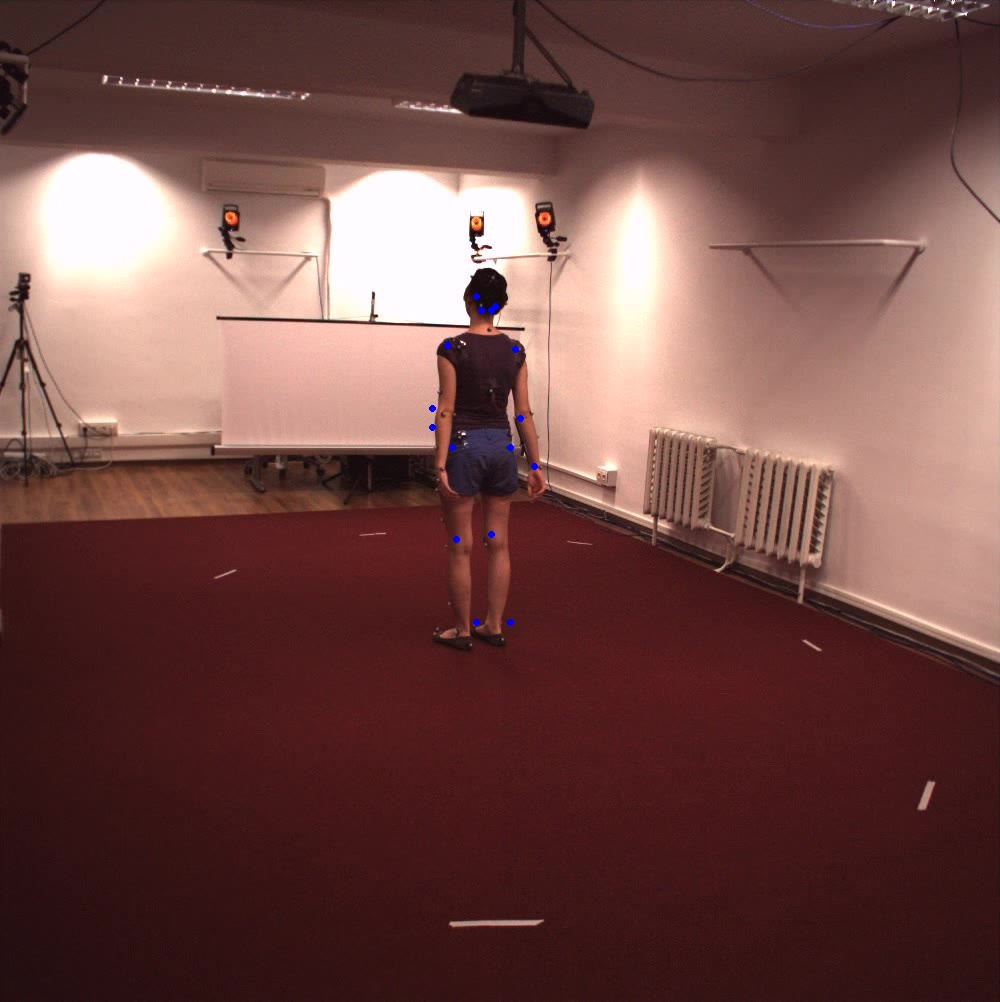### wduo commented Sep 25, 2019

 @Ixiaohuihuihui Hi, I test imgs use your codes. However the render results is bad. Do you know the reason of this cases? Thanks.

### Ixiaohuihuihui commented Sep 25, 2019

 @Ixiaohuihuihui Hi, I test imgs use your codes. However the render results is bad. Do you know the reason of this cases? Thanks. I don't know the special issuses. But I think maybe we should modify the parameters according to datasets. Which dataset images did you test on?

### carlottaruppert commented Sep 25, 2019

 @lxiaohuihuihui thanks so much for sharing! If I try with Coco data I get perfect results, but not on my own (because they are scaled differently?). Could you maybe explain what scale and pixel_std refer to exactly? I guess this is where it goes wrong. Thanks in advance!

### Ixiaohuihuihui commented Sep 25, 2019

 @lxiaohuihuihui thanks so much for sharing! If I try with Coco data I get perfect results, but not on my own (because they are scaled differently?). Could you maybe explain what scale and pixel_std refer to exactly? I guess this is where it goes wrong. Thanks in advance! Please refer: microsoft/human-pose-estimation.pytorch#26 (comment) Actually, I also don't know how to test in the wild image elegantly, but I guess maybe you can get the parameter by drawing a detection box manually or using fasterrcnn to detect the people. Your image size should be consistent wit the reference image in coco.

### carlottaruppert commented Sep 25, 2019

 I actually use detection bounding boxes from Mask RCNN and with coco data it works, I also checked wether the bboxes are correct and they are. Thanks anyway :)

### carlottaruppert commented Sep 26, 2019

 As mentioned in microsoft/human-pose-estimation.pytorch#26 (comment) the error was due to this line: c, s = _box2cs(box, data_numpy.shape, data_numpy.shape) instead it should be: c, s = _box2cs(box, data_numpy.shape, data_numpy.shape) So image_width and image_height were basically switched. I guess it worked better for Coco data, because the images are a lot more symmetrical than mine.

### tengshaofeng commented Oct 9, 2019 • edited

 @leoxiaobin @lxiaohuihuihui @MassyMeniche @jinfagang @wait1988 @njustczr i have try the inference for a single image. But I don not think the result is good. Is it the problem of trained model , or my inference code?the result is as follows: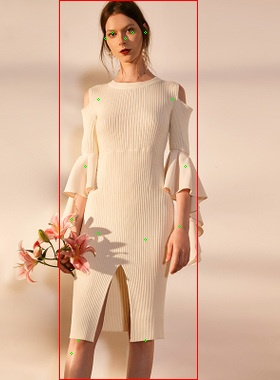### carlottaruppert commented Oct 9, 2019 • edited

 @tengshaofeng I'm not getting perfect results on your data either. I used w48_384x288 on your data I think it's because the joints of your humans are occluded by kinda baggy clothes and pose estimation is very sensitiv to that. But at least for the first picture it should work. It's just like that because my human detector did not work perfectly as you can see.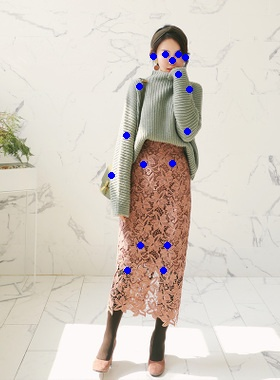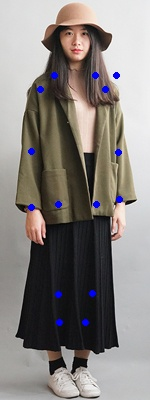but for example if I try on random data, where you can see the body parts better it works:

### tengshaofeng commented Oct 10, 2019 • edited

 @carlottaruppert , thanks so much for your reply. I think your performance is better than mine. Have u used the operation of fliping when test?

### carlottaruppert commented Oct 10, 2019

 As mentioned in microsoft/human-pose-estimation.pytorch#26 (comment) the error was due to this line: c, s = _box2cs(box, data_numpy.shape, data_numpy.shape) instead it should be: c, s = _box2cs(box, data_numpy.shape, data_numpy.shape) So image_width and image_height were basically switched. I guess it worked better for Coco data, because the images are a lot more symmetrical than mine. Have you done this? It is essantial. I haven't used flipping in testing, I am using a slightly altered version of the script posted in this issue.

### tengshaofeng commented Oct 10, 2019 • edited

 @carlottaruppert ,yes , I try as you said. It performance better. Really thanks for your advice. sorry to bother u again. the follow image is not good, can u try it for me?

### carlottaruppert commented Oct 10, 2019 • edited

 I think my result is better... since the picture width and height is basically the size of the bbox I scipped the Mask RCNN and coded the bbox hard. In addition I made sure that this part is commented out: if center != -1: scale = scale * 1.25 because HR Net enlarges the bbox and I didn't want that to happen because then it's bigger than the actual image and this can lead to errors. This could be your error too btw! I think it's confused by the dress again, so the legs aren't good

### tengshaofeng commented Oct 10, 2019

 @carlottaruppert , when i comment the "if center != -1: scale = scale * 1.25" the result is like follows: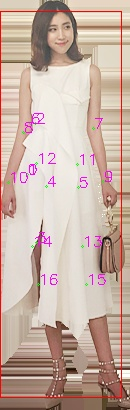maybe I should try the model of w48_384x288

### tengshaofeng commented Oct 10, 2019 • edited

 @carlottaruppert , when i set the box as large as the image, and I use the w48_384x288,but the result is as follows, I do not know why I can not get your result.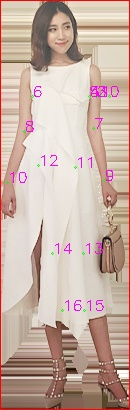can u share your inference code with me?

### carlottaruppert commented Oct 10, 2019 • edited

 from future import absolute_import from future import division from future import print_function import argparse import os import pprint import torch import torch.nn.parallel import torch.backends.cudnn as cudnn import torch.optim import torch.utils.data import torch.utils.data.distributed import torchvision.transforms as transforms import _init_paths from config import cfg from config import update_config from core.loss import JointsMSELoss from core.function import validate, get_final_preds from utils.utils import create_logger from utils.transforms import * import cv2 import dataset import models import numpy as np def parse_args(): parser = argparse.ArgumentParser(description='Train keypoints network') # general parser.add_argument('--cfg', help='experiment configure file name', default='experiments/mpii/hrnet/w32_256x256_adam_lr1e-3.yaml', type=str) ``````parser.add_argument('opts', help="Modify config options using the command-line", default=None, nargs=argparse.REMAINDER) parser.add_argument('--img-file', help='input your test img', type=str, default='') # philly parser.add_argument('--modelDir', help='model directory', type=str, default='') parser.add_argument('--logDir', help='log directory', type=str, default='') parser.add_argument('--dataDir', help='data directory', type=str, default='') parser.add_argument('--prevModelDir', help='prev Model directory', type=str, default='') args = parser.parse_args() return args `````` def _box2cs(box, image_width, image_height): x, y, w, h = box[:4] return _xywh2cs(x, y, w, h, image_width, image_height) def _xywh2cs(x, y, w, h, image_width, image_height): center = np.zeros((2), dtype=np.float32) center = x + w * 0.5 center = y + h * 0.5 ``````aspect_ratio = image_width * 1.0 / image_height pixel_std = 200 if w > aspect_ratio * h: h = w * 1.0 / aspect_ratio elif w < aspect_ratio * h: w = h * aspect_ratio scale = np.array( [w * 1.0 / pixel_std, h * 1.0 / pixel_std], dtype=np.float32) # if center != -1: # scale = scale * 1.25 return center, scale `````` def main(): args = parse_args() update_config(cfg, args) ``````logger, final_output_dir, tb_log_dir = create_logger( cfg, args.cfg, 'valid') logger.info(pprint.pformat(args)) logger.info(cfg) # cudnn related setting cudnn.benchmark = cfg.CUDNN.BENCHMARK torch.backends.cudnn.deterministic = cfg.CUDNN.DETERMINISTIC torch.backends.cudnn.enabled = cfg.CUDNN.ENABLED model = eval('models.'+cfg.MODEL.NAME+'.get_pose_net')( cfg, is_train=False ) if cfg.TEST.MODEL_FILE: logger.info('=> loading model from {}'.format(cfg.TEST.MODEL_FILE)) model.load_state_dict(torch.load(cfg.TEST.MODEL_FILE), strict=False) else: model_state_file = os.path.join( final_output_dir, 'final_state.pth' ) logger.info('=> loading model from {}'.format(model_state_file)) model.load_state_dict(torch.load(model_state_file)) model = torch.nn.DataParallel(model, device_ids=).cuda() # define loss function (criterion) and optimizer criterion = JointsMSELoss( use_target_weight=cfg.LOSS.USE_TARGET_WEIGHT ).cuda() # Loading an image image_file = args.img_file data_numpy = cv2.imread(image_file, cv2.IMREAD_COLOR | cv2.IMREAD_IGNORE_ORIENTATION) if data_numpy is None: logger.error('=> fail to read {}'.format(image_file)) raise ValueError('=> fail to read {}'.format(image_file)) # object detection box box = [0, 0, data_numpy.shape, data_numpy.shape] c, s = _box2cs(box, data_numpy.shape, data_numpy.shape) r = 0 trans = get_affine_transform(c, s, r, cfg.MODEL.IMAGE_SIZE) input = cv2.warpAffine( data_numpy, trans, (int(cfg.MODEL.IMAGE_SIZE), int(cfg.MODEL.IMAGE_SIZE)), flags=cv2.INTER_LINEAR) transform = transforms.Compose([ transforms.ToTensor(), transforms.Normalize(mean=[0.485, 0.456, 0.406], std=[0.229, 0.224, 0.225]), ]) input = transform(input).unsqueeze(0) # switch to evaluate mode model.eval() with torch.no_grad(): # compute output heatmap output = model(input) preds, maxvals = get_final_preds(cfg, output.clone().cpu().numpy(), np.asarray([c]), np.asarray([s])) image = data_numpy.copy() for mat in preds: x, y = int(mat), int(mat) cv2.circle(image, (x, y), 2, (255, 0, 0), 2) # vis result cv2.imwrite("test_h36m.jpg", image) cv2.imshow('res', image) cv2.waitKey(10000) `````` if name == 'main': main() Maybe your box format is not as it should be (x, y, width, height)? This is the version without the Mask RCNN annotation reading. and I'm calling it like this: python /tools/demo.py --cfg /experiments/coco/hrnet/w48_384x288_adam_lr1e-3.yaml --img-file 1.jpg TEST.MODEL_FILE /models/pytorch/pose_coco/pose_hrnet_w48_384x288.pth

### carlottaruppert commented Oct 10, 2019

 if you are using Mask RCNN as well, change the bbox format with this function: def change_box_to_coco_format(mask_box): """mask rcnn box structure looks as follows: y1,x1,y2,x2 where y1,x1 refer to the left upper coordinates and y2,x2 to the right lower coordinates of the bbox. coco however expects boxes in this format: x,y, width, height where x, y, refers to the left upper coordinates of the bbox.""" ``````coco_box = [0,0,0,0] coco_box=mask_box coco_box=mask_box coco_box= mask_box-mask_box coco_box= mask_box-mask_box return coco_box ``````

### tengshaofeng commented Oct 10, 2019

 @carlottaruppert , thanks so much.

### tengshaofeng commented Oct 10, 2019

 @carlottaruppert ， I found the problem. because of the bbox, my bbox is [0, 0, 130, 410], yours is [0, 0, 410, 130 ], the input image' width is 130, height is 410. as u said "Maybe your box format is not as it should be (x, y, width, height)" , i think your box is wrong, but I don't know why with your box the key points is right.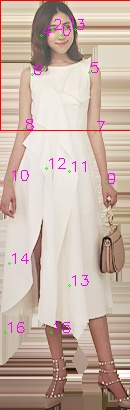### tengshaofeng commented Oct 11, 2019

 @carlottaruppert , I have found the solution after I read the code carefully. Actually，it shoud be: c, s = _box2cs(box, cfg.MODEL.IMAGE_SIZE, cfg.MODEL.IMAGE_SIZE) instead of: c, s = _box2cs(box, data_numpy.shape, data_numpy.shape)

### carlottaruppert commented Oct 11, 2019

 @tengshaofeng thank you so much! You're right! Don't know how I could miss that and no idea why it almost worked for me...

### eng100200 commented Oct 12, 2019

 @carlottaruppert hello, can i ask you some details?

### eng100200 commented Oct 14, 2019

 @carlottaruppert how many datasets you used in training? I want to train for multi-person in indoor environment.

### carlottaruppert commented Oct 14, 2019

 @eng100200 I didn't train at all. I'm only using HRNet to label my data. Sorry, but I guess I cannot help you.

### eng100200 commented Oct 15, 2019

 @carlottaruppert so you have used the test code only. Which pre-trained model you have used coco or mpii?

### carlottaruppert commented Oct 15, 2019

 I used the test code, to check wether retina or mask rcnn works better as a human detector for HR net and now I'm simply using the demo code. I am using coco pre trained weights w48.

### eng100200 commented Oct 16, 2019

 @carlottaruppert ok, can you share your email with me？ My email is sm_adnan21@hotmail.com

Merged

### alex9311 commented Feb 3, 2020

 I would say this issue can be closed with #161 being merged

### PCRKTY commented Nov 3, 2020 • edited

 @Ixiaohuihuihui Hi, I test an image of MPII datasets using your codes. But it shows ``````File "tools/demo.py", line 128, in main raise ValueError('=> fail to read {}'.format(image_file)) ValueError: => fail to read 084761779.jpg `````` The command is: ``````python tools/demo.py --cfg experiments/mpii/hrnet/w32_256x256_adam_lr1e-3.yaml --img-file 084761779.jpg TEST.MODEL_FILE output/mpii/pose_hrnet/w32_256x256_adam_lr1e-3/final_state.pth `````` Do you know the reason for this case? Thanks.

### xuxiaoxxxx commented Mar 10, 2021

 @tengshaofeng thank you so much! You're right! Don't know how I could miss that and no idea why it almost worked for me... First of all thank you for the great work.Is it possible to directly input the intercepted body image into the demo function?The predited picture I get is poor，I do not konw why.

### sunmengnan commented Mar 15, 2021

 why pixel_std is equal to 200？

### abraraltaf92 commented Jan 12, 2022

 By refecence this code[https://github.com/microsoft/human-pose-estimation.pytorch/issues/26#issuecomment-447404791], I can get good result. Make a file in the tools folder, and name it as "demo.py" ``````from __future__ import absolute_import from __future__ import division from __future__ import print_function import argparse import os import pprint import torch import torch.nn.parallel import torch.backends.cudnn as cudnn import torch.optim import torch.utils.data import torch.utils.data.distributed import torchvision.transforms as transforms import _init_paths from config import cfg from config import update_config from core.loss import JointsMSELoss from core.function import validate, get_final_preds from utils.utils import create_logger from utils.transforms import * import cv2 import dataset import models import numpy as np def parse_args(): parser = argparse.ArgumentParser(description='Train keypoints network') # general parser.add_argument('--cfg', help='experiment configure file name', default='experiments/mpii/hrnet/w32_256x256_adam_lr1e-3.yaml', type=str) parser.add_argument('opts', help="Modify config options using the command-line", default=None, nargs=argparse.REMAINDER) parser.add_argument('--img-file', help='input your test img', type=str, default='') # philly parser.add_argument('--modelDir', help='model directory', type=str, default='') parser.add_argument('--logDir', help='log directory', type=str, default='') parser.add_argument('--dataDir', help='data directory', type=str, default='') parser.add_argument('--prevModelDir', help='prev Model directory', type=str, default='') args = parser.parse_args() return args def _box2cs(box, image_width, image_height): x, y, w, h = box[:4] return _xywh2cs(x, y, w, h, image_width, image_height) def _xywh2cs(x, y, w, h, image_width, image_height): center = np.zeros((2), dtype=np.float32) center = x + w * 0.5 center = y + h * 0.5 aspect_ratio = image_width * 1.0 / image_height pixel_std = 200 if w > aspect_ratio * h: h = w * 1.0 / aspect_ratio elif w < aspect_ratio * h: w = h * aspect_ratio scale = np.array( [w * 1.0 / pixel_std, h * 1.0 / pixel_std], dtype=np.float32) if center != -1: scale = scale * 1.25 return center, scale def main(): args = parse_args() update_config(cfg, args) logger, final_output_dir, tb_log_dir = create_logger( cfg, args.cfg, 'valid') logger.info(pprint.pformat(args)) logger.info(cfg) # cudnn related setting cudnn.benchmark = cfg.CUDNN.BENCHMARK torch.backends.cudnn.deterministic = cfg.CUDNN.DETERMINISTIC torch.backends.cudnn.enabled = cfg.CUDNN.ENABLED model = eval('models.'+cfg.MODEL.NAME+'.get_pose_net')( cfg, is_train=False ) if cfg.TEST.MODEL_FILE: logger.info('=> loading model from {}'.format(cfg.TEST.MODEL_FILE)) model.load_state_dict(torch.load(cfg.TEST.MODEL_FILE), strict=False) else: model_state_file = os.path.join( final_output_dir, 'final_state.pth' ) logger.info('=> loading model from {}'.format(model_state_file)) model.load_state_dict(torch.load(model_state_file)) model = torch.nn.DataParallel(model, device_ids=cfg.GPUS).cuda() # define loss function (criterion) and optimizer criterion = JointsMSELoss( use_target_weight=cfg.LOSS.USE_TARGET_WEIGHT ).cuda() # Loading an image image_file = args.img_file data_numpy = cv2.imread(image_file, cv2.IMREAD_COLOR | cv2.IMREAD_IGNORE_ORIENTATION) if data_numpy is None: logger.error('=> fail to read {}'.format(image_file)) raise ValueError('=> fail to read {}'.format(image_file)) # object detection box box = [450, 160, 350, 560] c, s = _box2cs(box, cfg.MODEL.IMAGE_SIZE, cfg.MODEL.IMAGE_SIZE) r = 0 trans = get_affine_transform(c, s, r, cfg.MODEL.IMAGE_SIZE) input = cv2.warpAffine( data_numpy, trans, (int(cfg.MODEL.IMAGE_SIZE), int(cfg.MODEL.IMAGE_SIZE)), flags=cv2.INTER_LINEAR) transform = transforms.Compose([ transforms.ToTensor(), transforms.Normalize(mean=[0.485, 0.456, 0.406], std=[0.229, 0.224, 0.225]), ]) input = transform(input).unsqueeze(0) # switch to evaluate mode model.eval() with torch.no_grad(): # compute output heatmap output = model(input) preds, maxvals = get_final_preds(cfg, output.clone().cpu().numpy(), np.asarray([c]), np.asarray([s])) image = data_numpy.copy() for mat in preds: x, y = int(mat), int(mat) cv2.circle(image, (x, y), 2, (255, 0, 0), 2) # vis result cv2.imwrite("test_h36m.jpg", image) cv2.imshow('res', image) cv2.waitKey(10000) if __name__ == '__main__': main() `````` The command is: `python tools/demo.py --cfg experiments/coco/hrnet/w32_384x288_adam_lr1e-3.yaml --img-file 000002.jpg TEST.MODEL_FILE models/pytorch/pose_coco/pose_hrnet_w32_384x288.pth`I just did what is stated above but I am getting the issue as : ": cannot connect to X server " .

### Ixiaohuihuihui commented Jan 12, 2022 via email

 Thank you, I have received the e-mail! I will reply as soon as possible——Linhui Dai